# 1st PUC Maths Question Bank Chapter 7 Permutations and Combinations

Students can Download Maths Chapter 7 Permutations and Combinations Questions and Answers, Notes Pdf, 1st PUC Maths Question Bank with Answers helps you to revise the complete Karnataka State Board Syllabus and score more marks in your examinations.

## Karnataka 1st PUC Maths Question Bank Chapter 7 Permutations and Combinations

Question 1.
State fundamental principle of counting (or multiplication principle) for
(i) two events,
(ii) three events.
(i) Fundamental principle for two events states that “If an event can occur in m different ways, following which another even occur in n different ways, then the total number of occurrence of the events in the given order is m x n.

(ii) Fundamental principle for three events states that, “If an event can occur in m different ways, following which second event occurs in n different ways, following third event can occur in p different ways, then the total number of occurrence of the events in the given order is

Question 2.
How many 3-digit numbers can be formed from the digits 1, 2, 3, 4 and 5 assuming that
(i) Repetition of the digits is allowed?
(ii) Repetition of the digits is not allowed?
Given digits are 1, 2, 3, 4, 5.
To find : number of 3-digit numbers,
(i) Repetition of digit is allowed.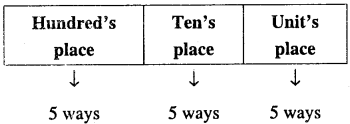Since repetition of digits is allowed, then each place can be filled by any of 5 given digits in 5 ways. Hence, three places can be filled in 5 x 5 x 5 ways i.e., 125 ways (By fundamental principle of counting). Required number of numbers = 125

(ii) Repetition of digit is not allowedSince the repetition of digits is not allowed then hundred’s place can be filled by any one of 5 given digits in 5 ways, ten’s place can be filled by any one of 4 left digits in 4 ways and unit’s place can be filled by any one of 3 left digits in 3 ways.
Hence, three places can be filled in
5 x 4 x 3 ways i.e., 60 ways.
∴ Required number of numbers = 60Question 3.
How many 3-digit even numbers can be formed from the digits 1, 2, 3, 4, 5, 6 if the digits can be repeated?
Given digits are 1, 2, 3, 4, 5, 6
To find : number of 3-digit even numbers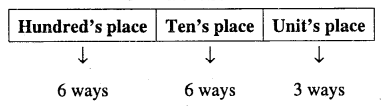Since the required number is even, it has to be end with 2, 4 or 6, therefore unit’s place can be filled in only 3 ways.

Since the repetition of the digits is allowed, therefore hundred’s place and ten’s place can be filled each in 6 ways.

Hence three places can be filled in 6 x 6 x 3 ways i.e., 108 ways.
∴ Required number of numbers = 108

Question 4.
How many 4-letter code can be formed using the first 10 letters of English alphabet, if no letter can be repeated?
Given : First 10 letters of English alphabet
To find : number of 4-letter codes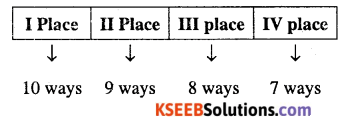Since required is 4-letter code, therefore, I-place can be filled in 10 ways, H, III and IV places can be filled in 9 ways, 8 ways and 7 ways respectively (because repetition of letter is not allowed).

Hence four places can be filled in
10 x 9 x 8 x 7 ways
i.e., 5040 ways
∴ Required number of 4-letter code = 5040

Question 5.
How many 5-digit telephone numbers can be constructed using the digits 0 to 9 if each number starts with 67 and no digit appears more than once?
Given : 0 to 9 digits (i.e., 10 digits)
To find : number of 5-digit telephone numbers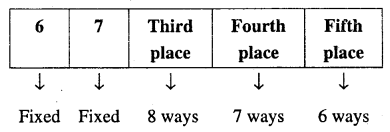There are 10 digits form 0 to 9 out of which 6 and 7 are fixed in first and second places respectively. Since the repetition of digits is not allowed, therefore the third, fourth and fifth places can be filled in 8, 7 and 6 ways respectively.

Hence five places can be filled in 8 x 7 x 6 ways i.e., 336 ways
Required number of telephone numbers = 336Question 6.
A coin is tossed 3 times and the out comes are recorded. How many possible outcomes are there?
When a coin is tossed once, there are two possible out comes head or tail when a coin is tossed three times, each time there are two possible out comes
Hence total number of out comes =2 x 2 x 2 = 8 .
Note: Possible out comes are {HHH, HHT, HTH, HIT, THH, THT, TTH, TIT}

Question 7.
Given 5 flags of different colours, how many different signals can be generated if each signal requires the use of 2 flags, one below the other?
Given : 5 different colour flags.
To find: Number of different signals, using 2 flagsWe have to select two flags one after the other. Therefore the upper flag (first flag) can be selected in 5 ways. Since both flags must be different colour, lower flag (second flag) can be selected in 4 ways.
∴ Number of different signals = 5 x 4 = 20

Question 8.
Find the number of 4 letter words, with or without meaning, which can be formed out of the letters of the word ROSE, where the repetition of the letters is not allowed.
Given work is ROSE
To find : Number of 4 letter words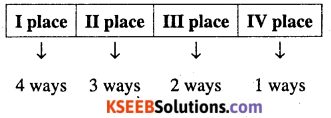Since required is 4 letter word in which repetition of letter is not allowed, therefore first place can be filled in 4 different ways and second, third and fourth places can be filled in 3 ways, 2 ways and 1 way respectively.
Hence four places can be filled in 4 x 3 x 2 x 1 ways
i.e., 24 ways
∴ Required number of words = 24Question 9.
Given 4 flags of different colours, how many different signals can be generated, if a signal requires the use of 2 flags one below the other.
Given : 4 flags of different colours
To find : Number of different signals, using 2 flagsTherefore the first flag can be selected in 4 ways.
Since both flags must be different colour, second flag can be selected in 3 ways . Number of different signals = 4 x 3 = 12

Question 10.
How many 2-digit even numbers can be formed from the digits 1, 2, 3, 4, 5 if the digits, can be repeated?
Given : 1, 2, 3, 4, 5 digits (5 digits)
To find : 2-digit even numbers∴ Required 2- digits even number
= 5 x 2 = 10

Question 11.
Find the number of different signals that can be generated by arranging at least 2 flags in order (one below the other) on a vertical staff, if five different flags are available.
Given : 5 different flags.
To find : Number of different signals
Signals that can be generated by arranging at least 2 flags means a signal can consist of either 2 flags, 3 flags, 4 flags or 5 flags.

Case (I):
Signals consisting of 2 flags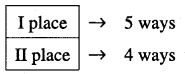We have to choose two flags one after the other. Therefore the first place can be filled in 5 ways and then the second place can be filled in 4 ways. Number of different signals that can be formed = 5 x 4 = 20

Case (II):
Signals consists of 3 flags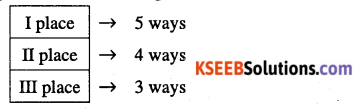∴ Required number of different signals can be formed = 5 x 4 x 3 x = 60

Case (III):
Signals consisting of 4 flags∴ Required number of different signals can be formed = 5 x 4 x 3 x = 120

Case (IV):
Signals consisting of 5 flags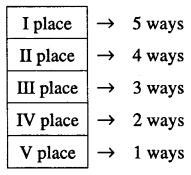∴ Required number of different signals can be formed = 5 x 4 x 3 x 2 x 1= 120
Therefore,total number of signals = 20 + 60 + 120 + 120 = 320

Permutations:

Question 12.
Define permutations.
Permutation is an arrangement in definite order of a number of objects taken some or all at a time.

Observations:

1. The number of permutations of n different objects taken r at a time, where 0<r<n and the objects do not repeat is
n(n-1)(n – 2)…… (n – r +1)
which is denoted by nPr
i.e., nPr= n(n – 1)(n – 2)……… (n – r +1)

2. The number of permutations of n different objects taken r at a time where repetition is allowed in nr.

3. Factorial rotation :
The notation n! represents the product of first n natural numbers.
i.e., n! = 1 x 2 x 3 x…… .x(n-1) x n

Remark:

(i) 0! = 1,1 x 2 = 2!, 1 x 2 x 3 = 3!
And so on.

(ii) n! = n(n-1)!
= n(n – 1)(n – 2)! (n≥2)
= n(n – 1)(n – 2)(n – 3)! (n≥3)

4. Formula for nPr
$$P_{r}=\frac{n !}{(n-r) !}, 0 \leq r \leq n$$
Note : nPn =n! and nPa =1

5. The number of permutations of n objects, where p objects are of the same kind and rest are all different is equal to

6. The number of permutations of n objects, where px objects are of one kind, p2 are of second kind,….. pk are of kth kind and the rest, if any, are of different kind is
$$\frac{n !}{p_{1} ! p_{2} ! \ldots \ldots p_{k} !}$$Question 13.
Evaluate:
(i) 5!
(ii) 7!
(iii) 8!
(iv) 4! – 3!
(v) 7! – 5!
Ans:
(i) 5!  = 5 x 4 x 3 x 2 x 1 = 120
(ii) 7! = 1 x 2 x 3 x 4 x 5 x 6 x 7 = 5040
(iii) 8!  = 1 x 2 x 3 x 4 x 5 x 6 x 7 x 8 = 40320
(iv) 4! – 3! = (4 x 3 x 2 x 1) – (3 x 2 x 1) = 24-6= 18
(v) 7! – 5! = (1 x 2 x 3 x 4 x 5 x 6 x 7) -(1 x 2 x 3 x 4 x 5) = 5040 – 120 = 4920

Question 14.
Compute:
(i)$$\frac{7 !}{5 !}$$
(ii)$$\frac{8 !}{6 ! \times 2 !}$$
(iii) $$\frac{12 !}{10 ! \times 2 !}$$Question 15.
Find x,if
(i) $$\frac{1}{6 !}+\frac{1}{7 !}=\frac{x}{8 !}$$
(ii)$$\frac{1}{8 !}+\frac{1}{9 !}=\frac{x}{10 !}$$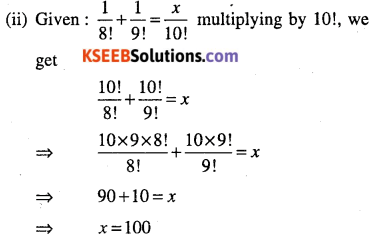Question 16.
Evaluate :
$$\frac{n !}{(n-r) !}$$, when
(i) n=5,r=2
(ii) n=6,r=2
(iii) n=9,r=5Question 17.
Evaluate :
$$\frac{n !}{r !(n-r) !}$$,When n=5, r=2.Question 18.
Find n, if
(i) $$P_{s}=42^{n} P_{3}, n>4$$
(ii) $$\frac{^{n} P_{4}}{^{n-1} P_{4}}=\frac{5}{3}, n>4$$
(iii) $$^{n-1} P_{3}:^{n} P_{4}=1: 9$$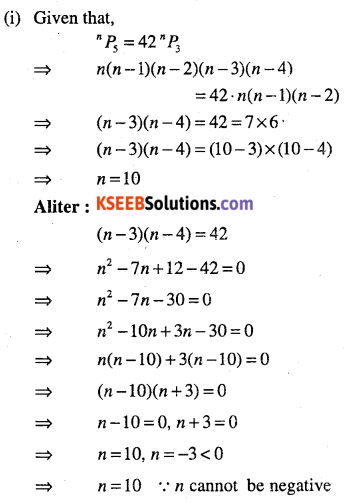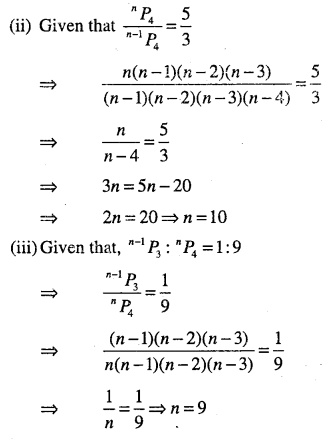Question 19.
Find r, if
(i) $$5 \cdot^{4} P_{r}=6 \cdot^{5} P_{r-1}$$
(ii) $$^{5} P_{r}=2 \cdot^{6} P_{r-1}$$
(iii) $$^{5} P_{r}=^{6} P_{r-1}$$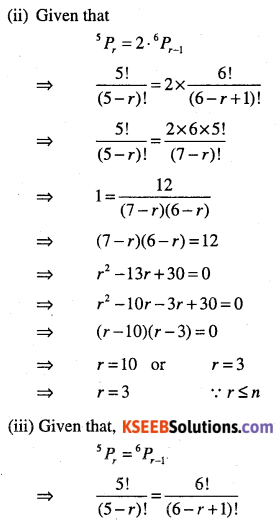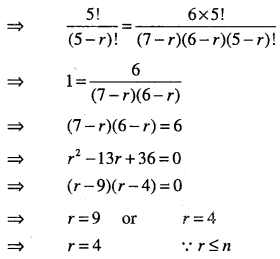Question 20.
How many 3-digit numbers can be formed by using the digits 1 to 9 if no digit is repeated?
The required number of numbers is equal to the number of ways in which 9 digits (all different) can be arranged taken 3 at a time. This can be done in 9P3 ways.
∴ Required number of numbers =  9P3
= 9 x 8 x 7 = 504Question 21.
How many four-digits numbers are there with no digit repeated?
Given : 0 to 9 digits (10 digits)
To find : 4-digit numbers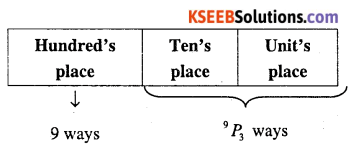First, thousand’s place on be filled by 9 ways from any one of the 1 to 9 digits (v ‘0’ (zero) is not placed in thousand’s place).
Then the remaining three places can be filled with the remaining 9 digits in 9P3 ways.
∴ Required number of numbers = 9 x 9P3
= 9 x (9 x 8 x 7)
= 4536

Question 22.
How many three-digit even numbers can be made using the digits 1, 2, 3, 4, 6, 7 if no digit is repeated?
Given : 1, 2, 3, 4, 6, 7 digits.
To find : 3-digit even numbers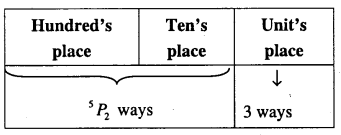Since required number is 3-digit even number, unit’s place can be filled in 3 ways from any one of the even digits 2, 4, 6. Then the remaining two places can be filled in 5 P2 ways.
∴ Required = 5P2 x 3 = (5 x 4) x 3 = 60

Question 23.
Find the number of 4-digit numbers that can be formed using the digits 1, 2, 3, 4, 5 if no digit is repeated. How many of these will be even?
Given : Digits 1, 2, 3, 4, 5 (5 digits)
To find :
(i) 4-digits numbers
(ii) 4-digit event numbers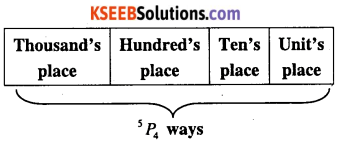The required number of numbers is equal to the number of ways in which 5 digits can be arranged taken 4 at a time. This can be done in SP4 ways.
∴ Required 4-digit n umber of numbers = 5P4 =5 x 4 x 3 x 2 = 120

(ii) To find the number of even numbers :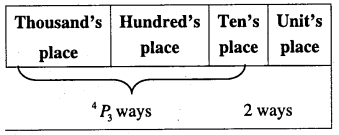In the given digits, even digits are 2 and 4 Since required word is 8 letters word, with or without meaning, therefore these 8 letters can be arranged in 4P3 ways.
∴ Required 4- digit number of numbers
2 x 4P3 =2 x (4 x 3 x 2) = 48

Question 24.
From a committee of 8 persons, in how many ways can we choose a chairman and a vice chairman assuming one person cannot hold more than one position?
Given : Number of persons in committee = 8
To find : Two positions (chairman, vice chairman)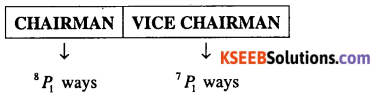Since one person cannot hold more than one position, therefore chairman post can be filled in 8P1 ways and vice chairman post can be filled in 7P1 ways.
∴ Required number of ways of choices =8P1 x 7P1
= 8 x 7 = 56

Question 25.
How many words, with or without meaning, can be formed using all the letters of the word EQUATION, using each letter exactly once?
Given : Work EQUATION (8 letters word)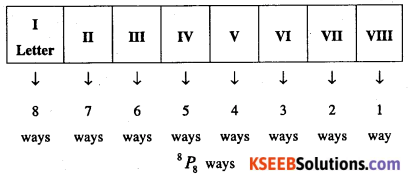Since required word is 8 letters word, with or without meaning, therefore these 8 letters can be arranged in 8P8 ways.
∴ Required number of words
= 8P8 = 8! = 40320Question 26.
How many words, with or without meaning can be made form the letters of the word MONDAY,assuming that no letter is repeated, if
(i) 4 letters are used at a time,
(ii) all letters are used at a time,
(iii) all letters are used but first letter is vowel?
Given work MONDAY has six different letters
(i) When only 4 letters are to be used, then the number of words formed
= 6 x 5 x 4 x 3 = 360

(ii) When all letters are to be used, then the number of words formed
= 6P6 =61 = 6 x 5 x 4 x 3 x 2 x 1 = 720

(iii) In the given word, vowels are A, OSince there are only two vowels A and O, therefore the first place can be filled in 2P1
(2 ways) ways and the remaining 5 places can be filled from remaining 5 letters,
in  5P5 ways.
∴ Required number of words
= 2P1 x 5P5
=2 x 5!= 2 x (5 x 4 x 3 x 2 x 1)
=2 x 120
=240

Question 27.
In how many of the distinct permutations of the letters in MISSISSIPPI do the four I’s not come together?
Given work is MISSISSIPPI, which has 11 letters of which M-1, S-4, I-4, P-2.
∴ Total number of arrangements
\begin{aligned} &=\frac{11 !}{4 ! 4 ! 2 !}\&=\frac{11 \times 10 \times 9 \times 8 \times 7 \times 6 \times 5 \times 4 !}{4 ! \times(4 \times 3 \times 2 \times 1) \times(2 \times 1)} \end{aligned}
To find : Number of permutations of the letters in which four I’s not come together.
First, we find number of permutations of the letters in which four I’s are kept together.Now, we treat four P s together as single object this single object together with 7 remaining objects (letters) will account for 8 objects. These 8 objects in which there are four 5’s and two P’s can be arranged in $$\frac{8 !}{4 ! 2 !}$$ ways.
∴ Number of words in which I’s kept together.Question 28.
In how many ways can the letters of the word PERMUTATIONS be arranged if,
(ii) vowels are all together
(iii) There are always 4 letters between P and 5
Ans:
In the word PERMUTATION, there are 12 letters of which T-2When P and S are fixed in first and last places, we have to arrange remaining
letters in 10 places can be done in $$\frac{10 !}{2 !}$$ ways
∴ Required number of words
$$\frac{10 !}{2 !}$$
$$=\frac{10 \times 9 \times 8 \times 7 \times 6 \times 5 \times 4 \times 3 \times 2 !}{2 !}$$
= 10 x 9 x 8 x 7 x 6 x 5 x 4 x 3
= 1814400(ii) Vowels in the given word are A, E, I, O, U (5 letters) and remaining letters are seven in which T’s are two.
Since vowels are all together, so we treat them as a single object this single object together with 7 remaining objects (letters) will account for 8 objects. These 8 objects can be arranged in $$\frac{8!}{2 !}$$ ways.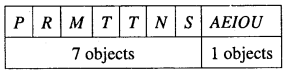Corresponding to each of these arrangements, 5 vowels can be rearranged in. 5! Ways
(5P5 ways)
∴ Required number of arrangements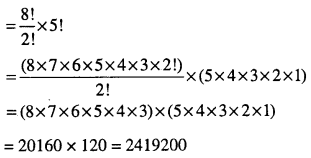(iii) Here, we want always 4 letters between P and S
∴ P and S can occupy 1st and 6th places
or 2nd and 7th places
or 3rd and 8th places
or 4th and 9th places
or 5th and 10th places
or 6th and 11th places
or 7th and 12th places
∴ P and S can be placed in 7 ways
Similarly S and P can be placed in 7 ways. The remaining 10 places can be filled with remaining 10 letters in $$\frac{8!}{2 !}$$ways (∵ T-2)
∴ Required arrangement
=7x2x$$\frac{8!}{2 !}$$
=7×10!=25401600

Question 29.
Find the number of different 8-letter arrangements that can be made from the letters of the word DAUGHTER so that
(i) all vowels occur together
(ii) all vowels do not occur together
Ans:
Given work DAUGHTER has 8 letters in which A, E,U are 3 vowels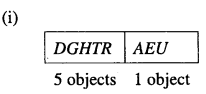We treat all vowels occur together as a single object and this single object together with 5 remaining objects (letters) will account for 6 object’s:’ These 6 objects can be arranged in 6P6 ways = 6! Ways corresponding to each of these arrangements, 3 vowels can be rearranged in 3! Ways (3 P3 ways)
∴ Required number of arrangements
= (6 x 5 x 4 x 3 x 2 x 1) x (3 x 2 x l)
= 720 x 6 = 4320

(ii) Required number of arrangements in which all vowels donot occur together = All possible arrangements of 8 letters taken all at time – number of arrangements in which the vowels occur together
= 8R8-6! x 3!
= 8! – 6! x 3!
= 61(8 x 7-3 x 2 x 1)
= 720 (50) = 36000

Question 30.
In how many ways can 4 red, 3 yellow and 2 green discs be arranged in a row if the discs of the same colour are indistinguishable?
Total number of discs = 4 + 3 + 2 = 9
Out of nine, 4 are of one king, 3 are of second kind and 2 are of third kind
∴ Required arrangements
$$=\frac{9 !}{4 ! 3 ! 2 !}$$
$$=\frac{9 \times 8 \times 7 \times 6 \times 5 \times 4 !}{4 ! \times(3 \times 2 \times 1) \times(2 \times 1)}$$Question 31.
Find the number of arrangements of the letters of the word In how many of these arrangement
(ii) do all the vowels always together
(iii) do the vowels never occur together
(iv) do the words begin with I and end in (Ex. No. 16. Text book)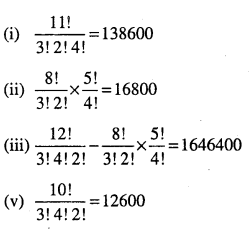Combination : Observations :

(i) The formula for finding the number of combinations ‘n’ different objects taken V at a time is nCr
(ii) In a combination order is not important
(iii) $$^{n} P_{r}=^{n} C_{r} \cdot r !, 0<r \leq n$$
i.e., total number of permutations of V different things (objects) taken V at a time is
nCr r!= nPrQuestion 1.
Show that nCr + nCTx = n+1Cr.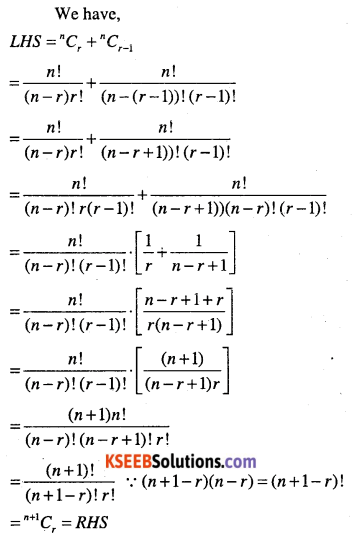Question 2.
$$\text { If }^{n} C_{8}=^{n} C_{2}, \text { find }^{n} C_{2}$$Question 3.
Determine
$$^{n} C_{17}, \text { if }^{n} C_{9}=^{n} C_{8}$$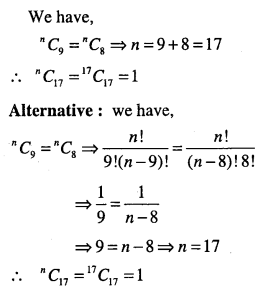Question 4.
Determine n if
(i) $$^{2 n} C_{3}:^{n} C_{3}=12: 1$$
(ii) $$^{2 n} C_{3}:^{n} C_{3}=11: 1$$Question 5.
How many chords can be drawn through 21 points on a circle?
We know that, chord is the line segment joining any two points on the circumference of a circle.
∴ Number of chords is equal to the number of ways in which we can choose 2 points out of 21 points.
∴ Required number of chords
= 21C2
$$=\frac{21 \times 20}{2 \times 1}=210$$Question 6.
In how many ways can a team of 3 boys and 3 girls be selected form 5 boys and 4 girls?
We have,
Number of girls = 4
Number of boys = 5
∴ 3 girls out of 4 girls can be selected in 4C3 ways and 3 boys out of 5 boys can be selected in 5C3 ways.
∴ Required number of ways
5C3 x 4C3
$$=\frac{5 \times 4 \times 3}{3 \times 2 \times 1} \times \frac{4 \times 3 \times 2}{3 \times 2 \times 1}$$
=10 x 4 = 40

Question 7.
Find the number of ways of selecting 9 balls from 6 red balls, 5 white balls and 5 blue balls if each selection consists of 3 balls of each choice?
We have
Number of red balls = 6
Number of White balls = 6
Number of blue balls = 6
Since each selection consists of 3 balls of each = 11  colours, so 3 red balls can be selected out of 6 balls in 6C3 ways, 3 white balls out of 5 can be selected in 5C3 ways and 3 blue balls out of 5 can be selected in 5C3 ways
∴ Required number of ways = 6C3 x 5C3 x 5C3
$$=\frac{6 \times 5 \times 4}{3 \times 2 \times 1} \times \frac{5 \times 4 \times 3}{3 \times 2 \times 1} \times \frac{5 \times 4 \times 3}{3 \times 2 \times 1}$$
= 20 x 10 x 10=2000

Question 8.
Determine the number of 5 cards combinations out of deck of 52 cards if there is exactly one ace is each combination.
We have,
Number of cards in a deck = 52.
If 52 cards, there are 4 aces and 48 other cards, (∵ 4 + 48 = 52).
If there is exactly one ace in each 5 card combination, then one ace out of 4 can be selected in 4C1 ways and 4 non-ace cards can be selected out of 48 in 48 C4 ways.
∴ Required number of combination = 4 C1 x 48C4
$$=\frac{4}{1} \times \frac{48 \times 47 \times 46 \times 45}{4 \times 3 \times 2 \times 1}=778320$$

Question 9.
What is the number of ways of choosing 4 cards from a pack of 52 playing cards? In how many of these
(i) four cards are of the same suit
(ii) four cards belong to four different suit
(iii) are face cards
(iv) two red cards and two are black card
(v) 4 cards are of the same colour.
We have,
Number of ways of choosing 4 cards from a pack of 52 cards is the number of combinations of 52 different things taken 4 at time
∴ Required number of ways = 52C4
$$=\frac{52 \times 51 \times 50 \times 49}{4 \times 3 \times 2 \times 1}=270725$$

(i) There are four suits, namely diamond, club, spade, heart and each suit has 13 cards. We have to choose 4 cards of the same suit so 4 diamond cards out of 13 diamond cards can be selected in 13C4 ways. Similarly,there are 13 C4 ways of choosing 4 spades and 13C4 ways of choosing 4 hearts.
∴ Required number of ways
= 13c4+13c4+13c4+13c4
$$=4 x^{13} C_{4}=4 \times \frac{13 \times 12 \times 11 \times 10}{4 \times 3 \times 2 \times 1}=2860$$

(ii) There are 13 cards in each suit we have to select 4 cards belonging to 4 different suits. There are l3C1 ways of choosing one card from 13 diamond cards, 13C1 ways of choosing 1 card from 13 cards of spades, 13C1 ways of choosing 1 card from 13 cards of club and 13C1 ways of choosing 1 card from 13 cards of hearts.
By multiplication principle, the required number of ways
= 13C1 x 13C1 x 3C1x 3C1
= 13 x 13 x 13 x 13 = 134 =28561(iii) Face cards means Kings, Queen, Jack there are 4 suits, each suit has 3 face cards. Therefore there are 12 face cards and 4 are to be selected out of 12 cards, in 12C4 ways
Required number of ways = 12C4
$$=\frac{12 \times 11 \times 10 \times 9}{4 \times 3 \times 2 \times 1}=495$$

(iv) There are 26 red cards and 26 black cards.
∴ We have select 2 red and 2 black cards. This can be done in
26C2 x 26C2 ways.
\begin{aligned} &=\frac{26 \times 25}{2 \times 1} \times \frac{26 \times 25}{2 \times 1}\\ &=325 \times 325=105625 \end{aligned}
∴ Required number of ways = 26C2 x 26C2
\begin{aligned}&=\frac{26 \times 25}{2 \times 1} \times \frac{26 \times 25}{2 \times 1}\\&=325 \times 325=105625\end{aligned}

(v) There are 26 red and 26 black cards
∴ 4 red cards can be selected in 26 C4 ways 4 black cards can be selected in 26 C4 ways
∴ Required number of ways = 26 C4 +26C4
$$=2 x^{26} C_{4}=\frac{26 \times 25 \times 24 \times 26}{4 \times 3 \times 2 \times 1}=29900$$Question 10.
In how many ways can one select a cricket team of eleven from 17 players in which only 5 players can bowl, if each cricket team of 11 must include exactly 4 bowlers?
We have,
Number of players = 17
Number of players to be select for team =11
Number of bowlers = 5
Number of bowlers to be select = 4
Number of players other than bowlers =12
4 bowlers out of 5 can be selected in 5C4 ways and 7(i.e., 11-4 = 7) other players can be selected out of 12 (i.e., 17 – 5 = 12) in 12C7 ways.
∴ Required number of ways = 5C4 x 12C7
$$=^{5} C_{1} \times^{12} C_{5} \quad ^{n} C_{r}=^{n} C_{n-r}$$
$$=5 \frac{12 \times 11 \times 10 \times 9 \times 8}{5 \times 4 \times 3 \times 2 \times 1}=3960$$

Question 11.
A bag contains 5 black and 6 red balls. Determine the number of ways which 2 black and 3 red balls can be selected.
2 black balls out of 5 can be selected in 5C2 ways and 3 red balls out of 6 can be selected in 6 C3 ways.
∴ Required number of ways = 5C2 x 6C3
$$=\frac{5 \times 4}{2 \times 1} \times \frac{6 \times 5 \times 4}{3 \times 2 \times 1}=200$$

Question 12.
In how many ways can be student choose a programme of 5 courses if 9 courses are available and 2 specific courses are compulsory for every student?
We have,
Number of available courses = 9
Number of compulsory courses = 2
∴ Number of remaining courses available for choice
=9-2=7
Number of courses to be choose = 5
But 2 courses are compulsory, so the student can select
3 courses out of remaining of courses in 7C3 ways.
Required number of ways = 7C3
$$=\frac{7 \times 6 \times 5}{3 \times 2 \times 1}=35$$

Question 13.
A committee of 3 persons is to be constituted from a group of 2 men and 3 women. In how many ways can this be done? How many of these committees should consists of 1 man and 2 women?
We have,
Number of men = 2
Number of women = 3
∴ Total number of persons = 2 + 3 = 5
Here, number of persons to be select = 3

(i) Required number of ways of selecting 3 persons
$$^{5} C_{3}=\frac{4 \times 5}{2}=10$$

Alternatives: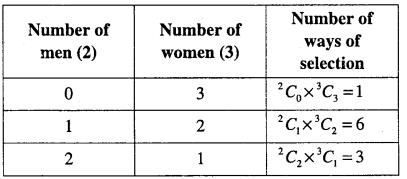(ii) 1 man can select out of 2 men in 2C, ways and 2 women can select out of 3 women in 3C2 ways.
∴ Required number of ways = 2C1 x 3C2 = 2 x 3 = 6

Miscellaneous Problems

Question 1.
How many words, with or without meaning, each of 2 vowels and 3 consonants can be formed from the letters of the word DAUGHTER?
Given word is DAUGHTER Here, vowels are A, E, U (3 in number)
Consonant are D, G, H, T, R (5 in number)
∴ 2 vowels out 3 and 3 consonant out of 5 can be selected in 3C2 x 5C3 ways and each
selection of 2 vowels and 3 consonants can be arranged in 5P5 =5! ways.
Required number of words = 3C2 x 5C3 x 5!
$$=3 \times \frac{5 \times 4}{2 \times 1} \times 120=3600$$Question 2.
How many words, with or without meaning can be formed using all the letters of the word EQUATION at a time so that the vowels and consonants occur together.
Given word is EQUATION
In this word, there are five voweIs A, E, I, O, U and three consonants Q, T, NQuestion 3.
A committee of 7 has to be formed from 9 boys and 4 girls. In how many ways can this be done when the committee consists of
(i) Exactly 3 girls ?
(ii) atleast 3 girls ?
(iii)atmost 3 girls
Number of girls = 4
Number of boys = 9
(i) Since the committee has to consists exactly 3 girls, the committee can consist of 3 girls and 4 boys.
∴ 3 girls out of 4 can be selected in 4C3 ways out of 4 boys out of 9 can be
selected in 9C4 ways
∴ Required number of ways = 4C3 x 9C4
$$=4 \times \frac{9 \times 8 \times 7 \times 6}{4 \times 3 \times 2 \times 1}=504$$

(ii) Since the committee has to consist at least 3 girls, the committee can consists of
(a) 3 girls and 4 boys
(b) 4 girls and 3 boys
(a) 3 girls and 4 boys can be selected in = 4C3 x 9C4 ways
(b) 4 girls and 3 boys can be selected in 4C4 x 9C3 ways
∴ Required number of ways
= 4C3 x 9C4 + 4C4 x 9C3
4 x 126 + 1 x 84 = 504 + 84 = 588

(iii) In this case, commitee may consists of 3 girls, 2 girls ,1 girl or no girl.Question 4.
If the different permutations of all the letter of the word EXAMINATION are listed as in a dictionary, how many words are there in this list before the first word starting with E?
We are required to find the number of permutations which begin with the letter A.
There are 11 letters in the given word of which A – 2, 1-2,N-2,
When A is fixed in the first place, remaining 10 $$\frac{10 !}{2 ! 2 !}$$letters can be arranged in ways.
∴ Required permulations
$$=\frac{10 \times 9 \times 8 \times 7 \times 6 \times 5 \times 4 \times 3}{2 \times 1}=907200$$

Question 5.
How many 6-digit numbers can be formed from the digits 0, 1, 3, 5, 7 and 9 which are divisible by 10 and no digit is repeated?
Given digits are 0, 1, 3, 5, 7, 9
Required number is 6 – digit which is divisible by 10.We know that, the number is divisible 10, the unit’s place should be compulsorily filled by 0. In 1P1 ways. The remaining 5 places can be filled in 5P5 ways.
= 5P5 x 1P1 =120 x 1= 120.

Question 6.
The English alphabet has 5 vowels and 21 consonants. How many words with two different vowels and 2 different consonants can be formed from the alphabet?Given: 5 vowels, 21 consonants
Required: Four letter words with 2 different vowels and 2 different consonants
∴ The number of ways of selecting 2 vowels out of 5= 5C2=10
The number of ways of selecting 2 consonants out of 21 =21C2 = 210
Number of combinations of 2 vowels and 2 consonants is 10 x 210 = 2100.
Now, each of these 2100 combinations has 4 letters which can be arranged themselves in 4P4 = 4! ways.
Required number of words = 2100 x 4!
= 2100 x 24 = 50400.Question 7.
In an examination, a question paper consists of 12 questions divided into two parts, part I and part II, containing 5 and 7 questions, respectively. A student is required to attempt 8 questions in all, selecting atleast 3 from each part. In how many ways can a student select the questions?
We have,
Number of questions in the question paper = 12
Number of questions in part 1 = 5
Number of question in part II = 7
Number of questions to attempt = 8
Condition: Selecting at least 3 from each part

 Part I (out of 5) Part II (out of 7) Number of ways 3 5 5C3 x 7C5 4 4 5C4 x 7C4 5 3 5C5 x 7C3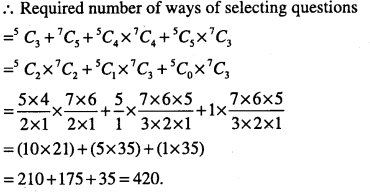Question 8.
Determine the number of 5-card combinations out of a deck of 52 cards if each selection of 5 cards has exactly one king?
We have to select one king out of 4 kings and four other cards out of 48. This can be done in 4C,x48C4 ways
$$=\frac{10 \times 9 \times 8 \times 7 \times 6 \times 5 \times 4 \times 3}{2 \times 1}=907200$$
∴ Required number of ways = 4C1 x 48C4
=4 x 2 x 47 x 46 x 45 = 778320

Question 9.
It is required to seat 5 men and 4 women in a row so that the women occupy the even places. How many such arrangements are possible?
We have,
Number of seats = 9Here, 4 even places and 5 odd places.
∴ 4 even places can be filled with 4 women in 4P4 = 4! ways and 5 odd places can be filled with 5 men in 5P5 =5! ways. Required number of ways
= 5! x 4!
= 120 x 24 = 2880

Question 10.
In how many ways can 5 girls and 3 boys be seated in a row so that no two boys are together?
First, we seat the 5 girls in 5! ways. For each such a arrangement, the three boys can be seated only at the ‘6’ cross marks places x G x G x G x G x G x Three boys can be selected in 6 P3 ways Hence total number of ways
= 5 x 6P3 = (5 x 4 x 3 x 2 x 1) x (6 x 5 x 4)
= 14400

Question 11.
In how many ways can be letters of the word ASSASSINATION be arranged so that all the S’s are together?
In the given word there are 13 letters of which S-4, A-3,1-2, N-2,0-1, T-l.
Since all four S’s are together then we treat them as a single object (letter). This single object (letter)
∴ These 10 items can be arranged in $$\frac{10 !}{2 ! 2 !}$$
∴ Required numbers of words
$$=\frac{10 \times 9 \times 8 \times 7 \times 6 \times 5 \times 4 \times 3}{2 \times 1}=907200$$

Question 12.
In how many words, with or without meaning, each of 3 vowels and 2 consonants can be formed from the letters of the word INVOLUTE?
In the word INVOLUTE there are 4 vowels (I, O, E, U) and 4 consonants (N, V, L, T)
Number of ways of selecting 3 vowels out of 4 = 4C3 = 4
Number of ways of selecting 2 consonants out of 4 = 4C2 =6
∴ Number of combinations of 3 vowels and 2 consonants is 4 x 6 = 24
Now, each of these 24 combinations has 5 letters Which can be arranged themselves in 5! Ways
∴ Required number words = 24 x 5 !
= 24 x 120 = 2880.Question 13.
A group consists of 4 girls and 7 boys. In how many ways can a team of 5 members selected if the team has
(i) no girl
(ii) at least 3 girls
(iii) at least one boy and one girl
(i) no girl
Table for computing the number of ways of selections.

 Girls (out of 4) Boys (out of 7) Number of ways 0 5 4C0 x 7C5

∴ Required number of ways = 4C0 x 7C5
$$=1 \times^{7} C_{2}=\frac{7 \times 6}{2 \times 1}=21$$

(ii) at least 3 girls

 Girls (out of 4) Boys (out of 7) Number of ways 3 2 4C3 x 7C2 4 1 4C4 x 7C1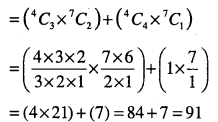(iii) at least one boy and one girl

 Girls (out of 4) Boys (out of 7) Number of ways 1 4 4C1 X 7C4 =140 2 3 4C2 x 7C3 = 210 3 2 4C3 x 7C2 =84 4 1 4C4 x 7C1 = 07

∴ Required numbers of ways
= 140 + 210+84 + 07 = 441

Question 14.
Find the number of words with or without meaning which can be made using all the letters of the word AGAIN. If these words are written as in a dictionary what will be the 50th word?
There are 5 letters in the word AGAIN, in which A – 2
∴ Required numbers of ways
$$=\frac{5 !}{2 !}=\frac{5 \times 4 \times 3 \times 2 \times 1}{2 \times 1}=60$$
To get the number of words starting with A, fix A in first place and remaining 4 letters can be arranged in 4! Ways = 24 ways
To get the number of word starting with G, fix G in first place and the remaining 4 letters in which A – 2
∴ Number of words starting with $$G=\frac{4 !}{2 !}=12$$
Similarly ,numbers of words starting with $$I=\frac{4 !}{2 !}=12$$
Total number of words so far obtained = 24 + 12 + 12 = 48
The 49th word is NAAGI and 50th word is NAAIGQuestion 15.
How many numbers greater than 1000000 can be formed by using the digits
1, 2,0,2,4,2,4?
Since the required numbers are greater than 1000000, then the number is begin with either 1,2 or 4
∴ Number of numbers begin with 1
$$=\frac{6 !}{3 ! 2 !}=\frac{6 \times 5 \times 4 \times 3 !}{3 !(2 \times 1)}=60$$

Total numbers beginning with 2
$$=\frac{6 !}{2 ! 2 !}=\frac{3 \times 4 \times 5 \times 6}{2}=180$$

Total numbers beginning with 4
$$=\frac{6 !}{3 !}=6 \times 5 \times 4=120$$

Required number of numbers
= 60+ 180 + 120 = 360

Alternative
Required number of numbers
$$=\frac{7 !}{3 ! 2 !}-\frac{6 !}{3 ! 2 !}=420-60=360$$

error: Content is protected !!